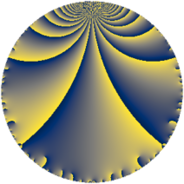Properties

 Label 42.3.gLevel $42$ Weight $3$ Character orbit 42.g Rep. character $\chi_{42}(19,\cdot)$ Character field $\Q(\zeta_{6})$ Dimension $4$ Newform subspaces $1$ Sturm bound $24$ Trace bound $0$

Related objects

Defining parameters

 Level: $$N$$ $$=$$ $$42 = 2 \cdot 3 \cdot 7$$ Weight: $$k$$ $$=$$ $$3$$ Character orbit: $$[\chi]$$ $$=$$ 42.g (of order $$6$$ and degree $$2$$) Character conductor: $$\operatorname{cond}(\chi)$$ $$=$$ $$7$$ Character field: $$\Q(\zeta_{6})$$ Newform subspaces: $$1$$ Sturm bound: $$24$$ Trace bound: $$0$$

Dimensions

The following table gives the dimensions of various subspaces of $$M_{3}(42, [\chi])$$.

Total New Old
Modular forms 40 4 36
Cusp forms 24 4 20
Eisenstein series 16 0 16

Trace form

 $$4 q + 6 q^{3} - 4 q^{4} + 12 q^{5} - 10 q^{7} + 6 q^{9} + O(q^{10})$$ $$4 q + 6 q^{3} - 4 q^{4} + 12 q^{5} - 10 q^{7} + 6 q^{9} - 24 q^{10} - 12 q^{11} - 12 q^{12} + 24 q^{14} + 24 q^{15} - 8 q^{16} - 48 q^{17} - 42 q^{19} + 24 q^{23} + 22 q^{25} + 96 q^{26} + 40 q^{28} - 24 q^{30} + 102 q^{31} - 36 q^{33} - 108 q^{35} - 24 q^{36} + 22 q^{37} + 24 q^{38} + 6 q^{39} + 48 q^{40} + 24 q^{42} + 28 q^{43} - 24 q^{44} + 36 q^{45} - 72 q^{46} - 132 q^{47} - 2 q^{49} - 192 q^{50} - 48 q^{51} + 12 q^{52} + 120 q^{53} - 48 q^{56} - 84 q^{57} - 96 q^{58} - 24 q^{59} - 24 q^{60} - 72 q^{61} + 30 q^{63} + 32 q^{64} + 180 q^{65} + 110 q^{67} + 96 q^{68} + 96 q^{70} + 312 q^{71} - 66 q^{73} - 48 q^{74} + 66 q^{75} + 120 q^{77} + 192 q^{78} - 10 q^{79} - 48 q^{80} - 18 q^{81} + 48 q^{82} + 60 q^{84} - 288 q^{85} - 24 q^{86} - 72 q^{89} - 222 q^{91} - 96 q^{92} + 102 q^{93} - 24 q^{94} - 132 q^{95} - 72 q^{99} + O(q^{100})$$

Decomposition of $$S_{3}^{\mathrm{new}}(42, [\chi])$$ into newform subspaces

Label Dim. $$A$$ Field CM Traces $q$-expansion
$a_{2}$ $a_{3}$ $a_{5}$ $a_{7}$
42.3.g.a $4$ $1.144$ $$\Q(\sqrt{2}, \sqrt{-3})$$ None $$0$$ $$6$$ $$12$$ $$-10$$ $$q+\beta _{1}q^{2}+(2+\beta _{2})q^{3}+2\beta _{2}q^{4}+(2+\cdots)q^{5}+\cdots$$

Decomposition of $$S_{3}^{\mathrm{old}}(42, [\chi])$$ into lower level spaces

$$S_{3}^{\mathrm{old}}(42, [\chi]) \cong$$ $$S_{3}^{\mathrm{new}}(14, [\chi])$$$$^{\oplus 2}$$$$\oplus$$$$S_{3}^{\mathrm{new}}(21, [\chi])$$$$^{\oplus 2}$$